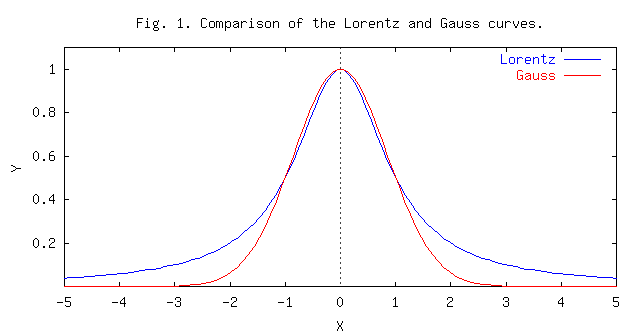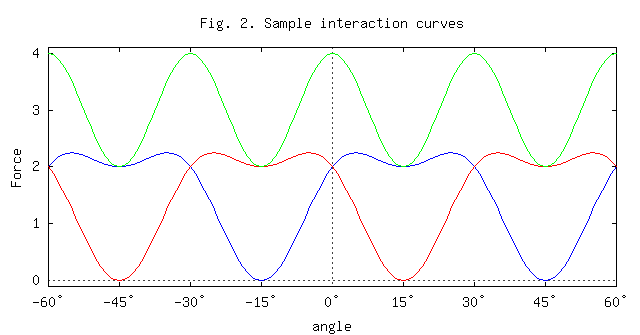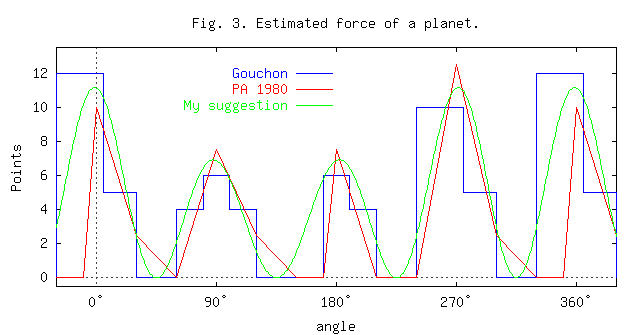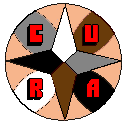The Manifesto | The Dominion | Texts and Articles | Review | Links | ACCUEIL (FR) | HOME (EN)

 Attempt of a scientific approach to astrology by Slawomir Stachniewicz (astrophysicist)

Introduction

Astrology is a very old domain of knowledge - it is at least a few thousand years old. Unfortunately, in the last two thousand years there was a stagnation. There were created a lot of theories without any verification and only recently there were some attempts to verify astrology using statistical methods. I have not heard about any trial to create a scientific theory of astrology - maybe this article may be a contribution to create such a theory. Apart from that I try to indicate what to pay attention to and what should be done first in such research.

Assumptions

I assume that general principles of the traditional astrology are in general O.K. (M. Gauquelin's research would suggest it). Another assumption is that astrology may be described using curves that are continous and their first derivative is also continous (it is some arbitrary assumption - not necessarily true but quite reasonable). Arguments of these functions are angles (because we look at the Celestial Sphere where we can describe positions using e.g. ecliptic longitudes and latitudes) so the best method of description are trigonometric functions.

Aspects

Aspects group around n/k * 360° so probably they are due to some resonance. Resonances are quite common in physics, e.g. in mechanics (waves etc.), celestial mechanics (so-called critical terms in perturbations), atomic and solid state physics. I think that planets interact with each other but this interaction is enormously strong around some values (resonance). It is not clear where this resonance comes from - I suppose it has something to do with waves and harmonics (1:1 or 360° - conjunction, 1:2 - opposition, 1:3 - trine etc.).

Now we have a good time to do mathematical description of these phenomena. Resonances may be described using intensity curves. Their shape is usually a Lorentz curve described by equation

y = a / ( 1 + (x/b)^2 )

where a and b describe intensity and width of the curve. Another common distribution is the Gaussian one described by equation

y = a * exp ( -1/2 (x/b)^2 )

but it is applied usually when describing random phenomena like errors distribution. If compared to the Lorentz curve its maximum is flatter but it goes to zero much faster for arguments distant from zero. Both distributions are shown at Fig. 1 where they are normalised in such a way that for x=0 y=1 and for x=+/-1 y=0.5 (the same intensity and the same width at half of intensity).Now let us think about the nature of this resonance. The situation is a bit complicated: we have not only intensity but also aspects are regarded as good, bad or neutral (I think good means less difficulties and bad more, the best situation is when there is more or less equilibrium between them - too much good aspects makes person to be lazy, he (or she) does not achieve anything significant in life or breaks down in case of difficulties). Of course, the strongest is the 1:1 resonance (conjunction) and it is neutral (the result depends on the nature of planets). 1:2 resonance (opposition) gives negative effects, 1:3 (trine) positive, 1:4 (or: double 1:2 or square) gives weaker effects than 1:2 but more negative etc. It seems that:

• 1:2 resonance is negative, 1:3 is positive, 1:5 is positive etc.
• the higher resonance the weaker influence (opposition is stronger than trine but weaker than conjunction etc.)
• putting resonances together weakens effect (square is weaker than opposition, sextile and nonile [40°] are weaker than trine)
• we can add and substract aspects and it affects them, e.g. quincunx (150=180-30) is approximately as strong as semisextile but is rather negative, sesquiquadrate (135=180-45=90+45) is similar to semisquare (attention, in this "theory" biquintile would be weaker than quintile and sesquiquadrate would be approximately as strong as semisquare but more negative etc.)
• aspect being a sum of two other ones is weaker but if it already exists, such operation can affect (strenghten or weaken) its influence - opposition is strenghtened by two squares but three sextiles and trine+sextile moderate it etc.
As we see, aspects would penetrate each other and description of their interaction (interference) would be complicated. Of course, e.g. for opposition influence from adding six semisextiles could be neglected. Apart from that, we could add and divide aspects non-stop and it would create some background - it would be possible to see effects of stronger aspects only, other ones could be very tiny. E.g. binonile (80°) could be very weak and it could be impossible to distinguish it from the background. Another effect could be that adding so many combinations of aspects could make these curves to look more like Gaussian than the Lorentz one. It is very complicated and even if we have a lot of empirical data it may be very difficult to construct a good theory.

Before we have a theory fully describing aspects I suggest following solution:

• above all, verify statistically meaning and force of aspects
• Introduce orbs for all aspects; if traditional data are O.K. it would be something like that: conjunction and opposition 9°, square and trine 7°, sextile 5° etc. (half of orb may be called half-width d)
• introduce force of aspect s depending on aspect and planets
• Influence of aspects could be described e.g. as s/(1+(dx/d)^2) where dx is a difference between theoretical length of aspect (e.g. 180° for opposition) and real difference of ecliptic longitudes. Of course, formula may look different but it would be necessary to collect a lot of data to verify that.
Of course, it would be an approximation only. Exact formula should be a combination of trigonometric functions.

Now I must ask a question: do aspect really depend on differences of ecliptic longitudes? Ecliptic is the plane of Earth's orbit around the Sun only. Maybe they do not depend on differences of ecliptic longitudes but real angular distances on the Celestial Sphere? For the Sun, the Moon and planets up to Neptune it would be almost the same - they are never far from the ecliptic (up to 5° for the Moon). There would be one important difference: in fact, no exact conjunctions and oppositions (even if ecliptic longitudes were the same there would be some difference in latitudes). Pluto would be in a different situation - its latitude can reach +/- 17° so in most cases (north or south latitudes greater than 10°) there would be no conjunctions and oppositions. Even worse with stars - only stars near ecliptic would be significant (conjunction!), e.g. Sirius (b=-39.6°) would be not significant. But there would be no problems with objects near ecliptic poles (no ecliptic longitude) - they would be in square to objects on ecliptic. There would be problems with traditional adding aspects - two exact sextiles might not to give an exact trine (it would be O.K. if they were coplanar with Earth).

I must ask some questions: does ecliptic have any meening apart from that planets' orbits are in its neighbourhood? Maybe we should take right ascensions instead of ecliptic longitudes? And why right ascensions and not galactic longitudes? What is the meaning (if any) of the Spring Equinox Point? What are Zodiac signs, what are their real boundaries, what is their influence's distribution? Some people claim that Zodiac are some energetic zones around Earth or that signs have some magnetic forces. So maybe we should look at magnetic poles instead of geographic ones? And what is the energy of these zones - connected with electromagnetic interactions? Strong? Weak? Gravitational? Electroweak? Maybe with so-called fivth force? We can exclude direct gravitational attraction (furniture's influence would be greater and, for example, Mercury's influence would be 6 mln. times lower than the Sun's one). I think we should not to talk about energies - we simply do not know how it works and some astrologers' speculations make increase existing chaos. The right answer could be given by scientific research only.

Influence of houses

There is no agreement about houses. Apart from the question: which system (a Polish astrologer, Kazimierz Urbanczyk, says that we should exclude equal houses, M-houses and Porphiry's system because they divide ecliptic instead of the Celestial Sphere - and I agree with him) it is not clear what is the role of the cusp of house. Is it the beginning (tradition), end (due Mrs. Schneider-Gauquelin ) or center. I have some idea how to obey this problem: cusps are the most important places in houses but they delimit influences of two houses. If so, the first house would have its traditional meaning but, maybe, would have some influence to the end of 12th house and the beginning of the 2nd one and a planet near the Ascendant would be stronger because of sum of influences of the 12th and 1st houses.

At Fig. 2 there is an example how can the interaction curves for houses look like. I defined a variable alpha = (n-1+x/d) * 30° where n denotes the house number, x is the distance from the cusp and d is the diameter of the house. Alpha shows the house and the position in the house where the body is. For Asc alpha=0, for IC alpha=90° etc. (for the cusp of n-th house alpha = (n-1) * 30°). More precisely, this formula is an approximation, exact calculation of alpha is complicated and depends on the house system.

Solid line denotes curves for odd houses, dotted line denotes curves for the even ones and the dashed-dotted line shows the sum of them. For the odd houses I took the function 1.5 + sin (6 alpha) + 0.5 cos (12 alpha), for the even ones the same function but with 30° shift in phase. Why such functions? First of all, they are a simple combination of trigonometric functions; moreover, they are almost constant entire the house; last but not least, out of the house they gradually decrease and they are zero in the half of the next (or previous) house. They are symmetric to the center of the house, the influence of the house starts in the half of the previous one and ends in the half of the next one. The sum is the greatest in the cusp and the lowest in the center of the house. In other words: in the middle the power of an object would be the weakest but the influence of the house would be the strongest. In the border between two houses the power of an object would be the strongest but it would be equal influence of both houses. But there is another question: what would be implications for the estimate of the strength of the planet?Of course, it can look different, it does not need to be symmetric, the influence of the next house can start in the last 5° of the house etc. - no problem, each integrable periodic function can be expanded using Fourier series (an trigonometric expansion, usually infinite).

I think that the Fourier expansion can be used as a temporary solution only - as a fit to experimental data. Exact solution should be a finite combination of trigonometric functions.

Some elements of such an analyse can be found e.g. at . The authors suggested some influence curve for an object in a house. Apart from that they suggest that influences of the houses interfere with each other. They say that similar (but not the same) story is with the influence of signs. However, I have some doubts. First of all, the authors did not write anything about how did they reach their results and did not provide any evidence (estimated after long research and use in practice - it is translation from the Polish translation). Moreover, their curves are continuous but they are not smooth (they have narrow points at the cusps of houses). In addition, they use so-called golden cut which refers to segments, not to angles. Such translation of geometric rules may be dangerous, one should apply them with a great care, as an approximation only. But maybe they are right.

Houses and dethronement of the ecliptic.

Next problem: what would be implication of the dethronement of the ecliptic? Borders of houses would depend not only on ecliptic longitudes but also on ecliptic latitudes. I was thinking what a reasonable house system should look like and it has lead me to the following assumptions:

• the plane of the horizon should be the border between the houses XII and I (in the East of the MC-IC line) and between the houses VI and VII (in the West of the MC-IC line)
• The plane of the meridian (South-Zenith-North-Nadir) should be the border between the houses IX and X (above the horizon) and between the houses III and IV (below the horizon)
• it should be as symmetric as possible.
The consequence is such a house system: its poles are North and South (in the horizon), the axis N-S is the common of six planes: horizon and next planes in 30° each - they would divide the sky into 12 equal regions and these regions would be the houses. The 1st house would be just below the eastern part of the horizon etc. It could be something like signs but it would be based on the N-S axis and the horizon. It is simply the Campanus system (let us stress that it depends on ecliptic latitudes!). Asc would be not a point - it would be a half of a great circle (more precisely: a half of the horizon). There would be a lot of problems with aspects to Asc and other houses - maybe it would be necessary to project planetary positions to the equatorial plane (perpendicular to the N-S axis) and to measure aspects on that plane? In plus: the planet in the 1st house could not be above the horizon (it can happen if we use traditional systems e.g. if we are in the Northern hemisphere and the planet has positive ecliptic latitude or vice versa). It can be true even if the ecliptic has really some meaning.

Next question: what happens if a planet (or another object) is in neighbourhood of one of the poles? I think that the further from the equator the weaker is the influence of the house - e.g. proportional to cos^2 b where b is the distance from the equator. In other words, the influence would be the strongest on the great circle drawn through the East, Zenith, West and Nadir and it would be the weakest (zero) at the poles or exactly in the North and South. I think similar story would be with the signs - it would decrease with the ecliptic latitude. But why? Because if we take an object in one of the ecliptic poles its ecliptic longitude is not defined so it does not belong to any sign. After an arbitrarily small shift (e.g. 0.01") it would be in one of the signs (or between two of them). It seems to make sense, in such case the influence of the signs should be minimal - in other case there would be discontinuities (or even singularities) on both poles.

Such a house system could be suitable even for the sub-polar zones. But what would happen in case that somebody is born exactly at a geographic pole? Or 0.01 mm from a pole? I assume that we can know poles positions with so good precision (in fact, the error is in order of 1 m). All meridians pass through poles so we cannot speak about the south direction because, in fact, any direction is south - and what about the MC determination? So influence of houses should depend on the geographic latitude (no influence at all at both poles ???!). By the way, it would be quite easy to verify: take two sets of people from near the equator (e.g. India) and a pole (e.g. Norway). If I am right the houses influence on planets for people from Norway should be much smaller.

One can ask Well, but how to understand decreasing influence of the signs and houses?. It is a very good question (next question, please). I suppose that objects near ecliptic poles would be more pure, less influenced by the sign's influence [it would not apply to the planets, e.g. Pluto's maximal distance from the ecliptic is about 17° , for other planets even less, so it would be difficult to catch such tiny effects] and for houses the effect would be that the planet (or other object) would have less influence to things governed by the house.

Of course, at the Southern hemisphere it would be opposite - there MC would be in the north; for the equator (in the tropical region, more precisely) MC would be near the Zenith. Next question: maybe MC is simply the ecliptic longitude of the zenith? Hmm, in such case MC would be exactly between Asc. and Dsc. so we would get equal houses 30° from Asc. and the local meridian would not play any role.

Force of the planets and their house positions

There are plenty methods to estimate force of the planets. I know two of them: published in the Problemy Astrologii 1980  and from the Dictionaire Astrologique by H. Gouchon . I paid attention especially to their house-dependent parts. Both methods are shown at Fig. 3 (dotted and solid lines) but in order to compare them better I divided the PA estimates by 2. The independent variable is alpha (already defined; for the cusps of the houses alpha = (n-1) * 30°).

In both methods we have two big peaks near Asc and MC (about 12 points) and two lower peaks near Dsc and IC (about 6 points), between them functions fall to zero. As we want to approximate that by smooth functions the best solution may be trigonomethric ones. Let us take as the first approximation

9/2 [cos (4 alpha) + 1] .

It is not a very good solution - we obtain four equal peaks 9 points each. So let us multiply that by the function that has a maximum between Asc and MC, a minimum between Dsc and IC and in the maximum is 2 times greater than in the minimum. Moreover, its mean value should be 1. Such function may be e.g.. 1/3 [3 + cos (alpha + 45°)] so we obtain the following function:

3/2 [cos (4 alpha) + 1] * [3 + cos (alpha + 45°)]

and it is also shown at Fig. 3 (green line). As we can see, it is a quite good approximation.The price for this approximation is some shift of peaks: due to additional factor peaks are not perfectly symmetric and are not placed exactly on the Asc, Dsc, MC and IC. But I think it may be O.K. as the first approximation.

Summary

I introduced some hyphotesis that try to make a link between the Science (especially Physics) and Astrology, explain some divergences between traditional astrology and results of empirical research and to establish a theoretical base for further research. All of them require to be verified using statistical methods. If we know better such elements as aspects and their interaction curves it will be possible to construct a theory that will describe them. Until we do not know the nature of astrological interactions they will be pure phenomenological i.e. describing phenomena only.

Bibliography

 Françoise Gauquelin, Tajemnica niezgodnosci tradycyjnej domifikacji z wynikami badan empirycznych wyjasniona? (The Mystery of the Disagreement Between Traditional Domification and Results of Empirical Research Explained? - a shortened translation of the article: The Greek Error or Return to Babylon, The Schneider-Gauquelin Research Journal, III.3, 1985). Problemy Astrologii (1986-1987), Stowarzyszenie Astrologow w Poznaniu.
 Bruno and Louise Huber, Psychologia astrologicznego systemu domow (Die astrologischen Häuser, Verlag Astrologisch-Psychologisches Institut Adliswill/Zürich, 1975), Studio Astropsychologii, 1995.
 Tadeusz Wrotkowski, Teoria dominanty (Theory of the dominant). Problemy Astrologii (1980), Stowarzyszenie Astrologow w Poznaniu.
 Henri J. Gouchon, Dictionnaire Astrologique, Paris, Dervy-Livres, 1986.